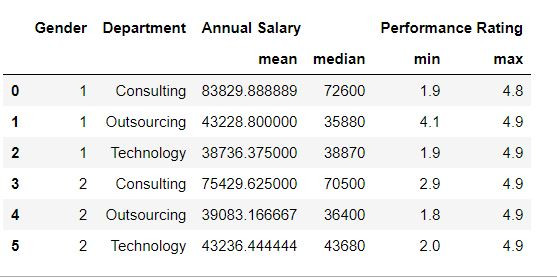Search
•Ekta Aggarwal

# Group by and aggregate functions in Python

In this tutorial we shall learn about how can we calculate summary statistics by grouping our data on the basis of categorical variables.

Following functions to obtain summary statistics are available in Python :### Dataset:

In this tutorial we will make use of following CSV file:

Employee_info
.csv

Let us read our file using pandas' read_csv function. Do specify the file path where your file is located:

```import pandas as pd

Task: Retrieve the minimum value for all the variables for each department.

Since we want to divide our data on the basis of each department and then get the minimum values, for this we have grouped our data by department and then applied the min( ) function to get minimum values for all the columns.

`mydata.groupby('Department').min()`### Grouping by multiple variables

Task: Retrieve the minimum value for all the variables for each department and gender.

Here have specified 2 variables Department and Gender in our group by

`mydata.groupby(['Department','Gender']).min()`By default group by is returning minimum values for all the columns. To fetch minimum values for a single column (say Annual Salary ), after group_by we select the column name and then calculate the summary statistic.

`mydata.groupby(['Department','Gender'])['Annual Salary '].min()`### Calculating multiple summary statistics:

Task: Calculate mean and median values for each Department and Gender combination.

To calculate multiple summary statistics we can use agg( ) function. Inside agg we have defined the summary statistics which we want to retrieve.

`mydata.groupby(['Gender','Department']).agg(['mean','median'])`Task: Calculate mean and median for annual salary and minimum and maximum performance rating for each Department and Gender.

Since we need to calculate different but multiple statistics for Annual Salary and Performance Rating, in the agg( ) function we have defined a dictionary:

In this dictionary keys are: The column names on which the calculation is to be done.

Values are: a list of summary statistics needed for the keys

```grouped = mydata.groupby(['Gender','Department'])\
.agg({"Annual Salary " : ['mean','median'], "Performance Rating" : ['min','max']})```

To rename the columns, we have saved our data in a new dataframe grouped and have renamed the column names as follows:

```grouped.columns = ['mean_salary','median_salary','min_rating','max_rating']
grouped```### as_index

By default the grouped variables are stored as indices in the resultant data. To store them as columns in the new data we define as_index = False in groupby.

```mydata.groupby(['Gender','Department'],as_index = False)\
.agg({"Annual Salary " : ['mean','median'], "Performance Rating" : ['min','max']})```Tags:

See All